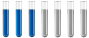## material balance

Chemistry and homework help forum.

Organic Chemistry, Analytical Chemistry, Biochemistry, Physical Chemistry, Computational Chemistry, Theoretical Chemistry, High School Chemistry, Colledge Chemistry and University Chemistry Forum.

Share your chemistry ideas, discuss chemical problems, ask for help with scientific chemistry questions, inspire others by your chemistry vision!

Please feel free to start a scientific chemistry discussion here!

Discuss chemistry homework problems with experts!

Ask for help with chemical questions and help others with your chemistry knowledge!

Moderators: expert, ChenBeier, Xen

555gag
Full MemberPosts: 13
Joined: Fri Mar 20, 2020 12:58 pm

### material balance

100 kg / h H2 is to be produced. The conversion rate reached is 85%. The reaction proceeds at
temperature 800 Â° C and pressure 202,6 kPa. The feed gases should be preheated from 200 Â° C. O2 contains
7% inert impurities and CH4 are dosed with a 5% molar excess. Draw the scheme
with substance and energy flows. Perform mass and volume balance a
energy balance process. (Neglect heat to heat dirt). Specify the conditions for
which process is used in industry (temperature, catalyst, pressure,).

my solution:

CH4 +1/2 O2 = CO + 2H2

we have a node where the current 1 - ch4 and the current 2 - o2, inert and the node outputs the current 3 - ch4, inert, o2, CO, H2
nH2 = mH2/MH2 = 100000/2,009 = 47 846 mol
nCO = nH2/2* = 23 923 mol
nCH4(surplus)= nH2/2 *100/85 * 1,05 = 29 551 mol
nO2=nH2/4 = 11961 mol

Current composition 3.
n(inert) = no2*5/85 = 11961*5/85 = 703,58 mol
nH2 = 47846 mol
nCO=23 923 mol
nCH4 = nch4(surplus) - 1/2*nH2 = 29551 - 0,5*47846 = 5628 mol
nO2 = 11 961 mol
I could ask for a check, I'm not sure that the conversion is counted correctly

I only deal with this part, I will calculate the rest then, and I do not want to count unnecessarily if I do not know the correct composition ...

Is it correct? If not, could I ask for an explanation? Thank you
ChenBeier
Distinguished MemberPosts: 436
Joined: Wed Sep 27, 2017 7:25 am
Location: Berlin, Germany
I think the calculation of oxygen has also considered by 85%, like you did for methane. Also the amount has to be increased, because of 7% impurities.
555gag
Full MemberPosts: 13
Joined: Fri Mar 20, 2020 12:58 pm
thank you so much, so now it's right?

Current composition 1
nO2=(nH2/4)*100/85= 14 072 mol

Current composition 3.
nO2 = 14 072 mol
n(inert) = no2*5/85 = 14 072*5/85 = 828 mol
ChenBeier
Distinguished MemberPosts: 436
Joined: Wed Sep 27, 2017 7:25 am
Location: Berlin, Germany
The inert I dont understand.

First I would calculate all at 100%. You did partly already.

100 kg H2 is 47846 mol
The reaction yield is 85%
So it will be 56289 mol in consideration.

The CO will be the half 28147 mol

The CH4 is the same 28147 plus 5% excess = 31099 mol
O2 will be 14072 mol, but 7% impurities its only 93% = so will need 15131 mol